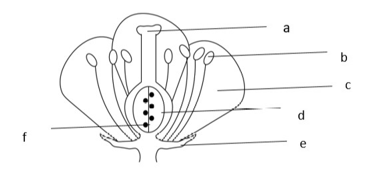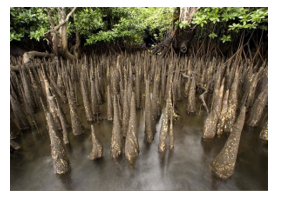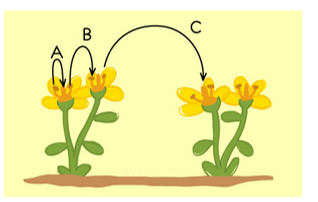# Important Questions for Getting to know plants

In this page we have Extra Questions for Class 6 Science Chapter 7 Getting to know plants . Hope you like them and do not forget to like , social share and comment at the end of the page.

Question 1
GIVE REASONS FOR THE FOLLOWINGS.
(i)Why plants like cactus develop spines?
(ii)‘Transpiration do not take place at night’.
(iii)Why leaves are green in colour?
(iv)‘Tap root is also known as true roots’
(v)Mango is known as a tree and not as shrub.

Question 2
HOTS
(i)Why complete flowers are also known as bisexual flower?
(ii)How do you think a huge banyan tree gets its support?
(iii)Why stems are modified in cactus?
(iv)Why do desert plants have relatively longer roots?
(v)Why grapes have tendrils?

## SKILL/DIAGRAM-BASED QUESTIONS.

Question 3(i). Name the parts labeled a-f
(ii). state the function of ‘c’
(iii). Is it a complete or incomplete flower?

Question 4(i)Identify the structure shown.
(ii)What is the other name used for this structure?
(iii)State the significance of this structure.
(iv)Where are these structures found?
(v)Is it a modified root or shoot?

Question 51. Identify the process shown.
2. Name the process labelled A, B, C.
3. List two agents that favours this process.

Question 6
(i)How do plants like Dodder obtain its nutrition?
(ii)What role does modified root in asparagus play?
(iii)Apart from the above mentioned modified functions of root state any two other functions of root?

Question 7
List the different functions of stem.### Practice Question

Question 1 What is $\frac {1}{2} + \frac {3}{4}$ ?
A)$\frac {5}{4}$
B)$\frac {1}{4}$
C)$1$
D)$\frac {4}{5}$
Question 2 Pinhole camera produces an ?
A)An erect and small image
B)an Inverted and small image
C)An inverted and enlarged image
D)None of the above